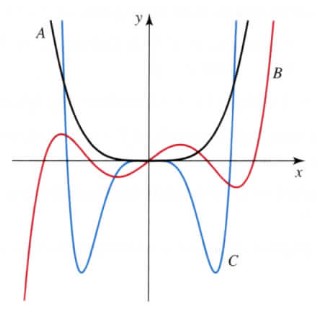×
Get Full Access to Calculus: Early Transcendentals - 1 Edition - Chapter 1.1 - Problem 53e
Get Full Access to Calculus: Early Transcendentals - 1 Edition - Chapter 1.1 - Problem 53e

×

# Symmetry i n graphs State whether the functionsISBN: 9780321570567 2

## Solution for problem 53E Chapter 1.1

Calculus: Early Transcendentals | 1st Edition

• Textbook Solutions
• 2901 Step-by-step solutions solved by professors and subject experts
• Get 24/7 help from StudySoup virtual teaching assistantsCalculus: Early Transcendentals | 1st Edition

4 5 1 422 Reviews
20
3
Problem 53E

Symmetry in graphs State whether the functions represented by graphs A, B, and C in the figure are even, odd, or neither.Step-by-Step Solution:

Step-by-step solution Step 1 of 5 We need to tell the given function graphs A,B and C are even, odd or neither. The function f(x) is even if f(-x) is equal to f(x) for all x in the domain. This means that function f(x) is symmetric about y-axis and we can say that the function values for x and -x are equal. Polynomials consisting of only even powers of the variable (of the form x , 2n where n is a non negative integer) are even functions.

Step 2 of 5

Step 3 of 5

## Discover and learn what students are asking

Statistics: Informed Decisions Using Data : Inference about the Difference between Two Medians: Dependent Samples
?Rat Hemoglobin Hemoglobin helps the red blood cells transport oxygen and remove carbon dioxide. Researchers at NASA wanted to discover the effects of

Unlock Textbook Solution